# Example of the method of locally updated planes¶

To run the code below:

1. Click on the cell to select it.
2. Press SHIFT+ENTER on your keyboard or press the play button () in the toolbar above.

For an overview of the notebook interface click on Help > User Interface Tour in the menu on the top.

## In this example, we find a reaction path on the Müller potential using the method of locally updated planes¶

• Ulitsky, A., & Elber, R. (1990). A new technique to calculate steepest descent paths in flexible polyatomic systems. The Journal of Chemical Physics, 92(2), 1510. doi:10.1063/1.458112

• Müller, K. (1980). Reaction paths on multidimensional energy hypersurfaces. Angewandte Chemie International Edition in English, 19(1), 1–13. doi:10.1002/anie.198000013

In :
%matplotlib inline
from potential import Potential
from path import Path

import numpy as np
import matplotlib.pyplot as plt


### First, we set up the potential energy function¶

In :
potential = Potential()
potential.plot()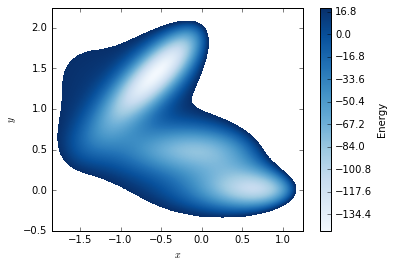### Next, we initialize the reaction path by declaring the location of the reactant and the product as well as the number of nodes in the path.¶

The variable threshold determines the convergence criterion for the method. The smaller its value, the longer the method will take to converge.

In :
reactant = np.array([0.623499, 0.028038])
product = np.array([-0.558233, 1.441716])
num_nodes = 10
threshold = 1e-4
path = Path(reactant, product, num_nodes, potential, threshold)
path.plot()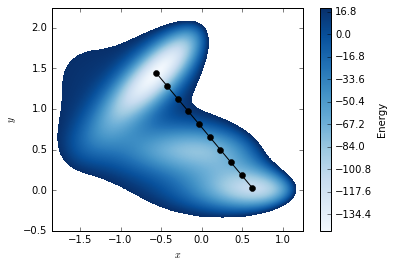### Finally, we iteratively refine the initial path until convergence is attained.¶

In :
for p in iter(path):
path.plot()
plt.show()

Current energy: -569.692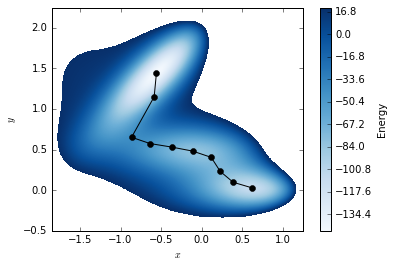Current energy: -626.678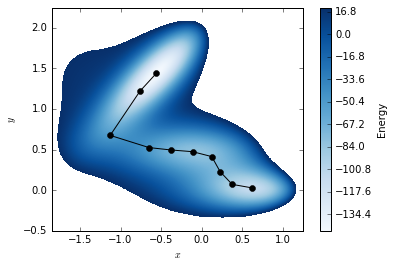Current energy: -627.712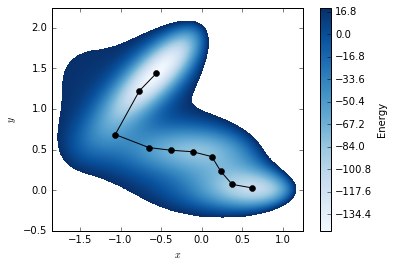Current energy: -627.721


## Another example joining two of the metastable states.¶

We add some random perturbation to the initial linear interpolation between the endpoints.

In :
reactant = np.array([0.623499, 0.028038])
product = np.array([0.0, 0.5])
num_nodes = 10
threshold = 5e-4
path = Path(reactant, product, num_nodes, potential, threshold)
# Add random noise to the initial, linear interpolant.
perturbation = 1e-1 * (2.0 * np.random.rand(num_nodes - 2, 2) - 1.0)
path.points[1:-1, :] += perturbation
path.plot()
plt.show()
for p in iter(path):
path.plot()
plt.show()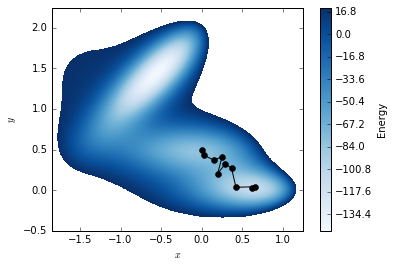Current energy: -680.023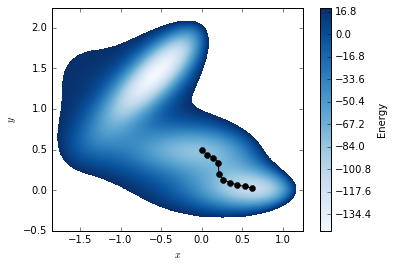Current energy: -682.783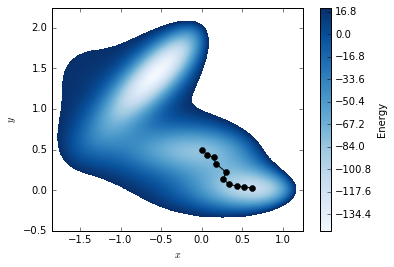Current energy: -686.903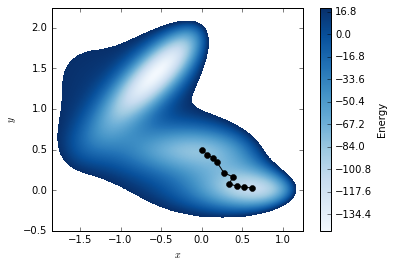Current energy: -691.551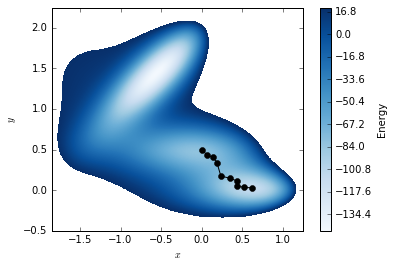Current energy: -696.878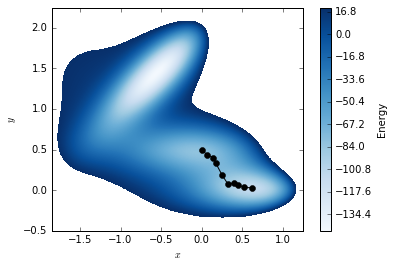Current energy: -699.338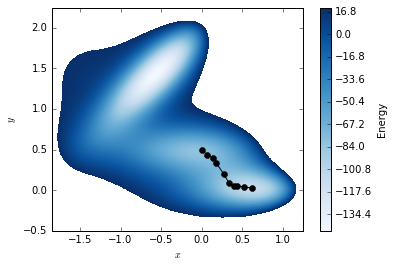Current energy: -700.14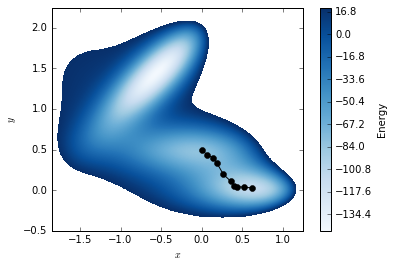Current energy: -700.568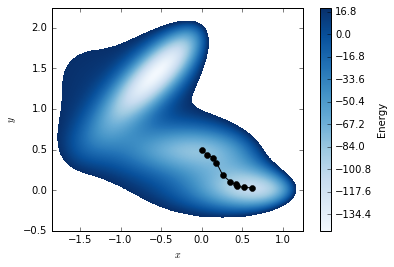Current energy: -700.781